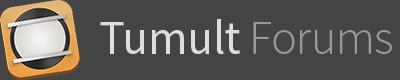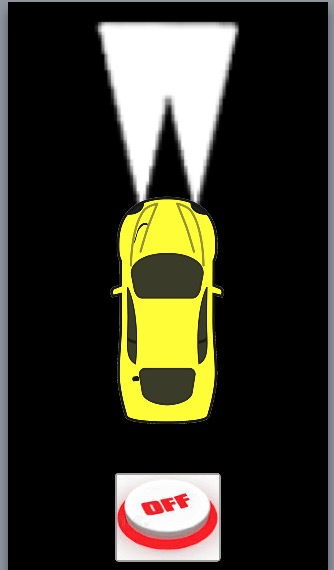In this example, the button On/Off is working as expected, but the light should be off when the file runs for the first time.
The JS was taken from another post in this forum.
Any hint?

This is very simple.

Put a rectangle in the place on the scene where turn_3.png (the headlight) is. Set the rectangle with no border and and no colour fill.

Remove turn_3.png from the scene but not the project resources .
Give the rectangle the id = turn_3

1 Like

@MarkHunte, I understand that, but there will be many light beams and it will cross each other, if I use rectangles the images will be one over the other and the illusion of crossing lights will be lost, I added a second example with the rectangles you suggested. if both lights are ON the small one does not show. So I am still struggling to make the lights show off at the start using only images.

Firstly, if you have images they will act the same as the rectangles.
The only difference here is the rectangle is blank at the start with no image!.

Secondly, How is that related to this question?

I know it is not always easy to determine when to start a new thread but If all these questions are actually related can you try and keep them in a single thread as it is getting confusing to work out what you are after in each.

Cheers

1 Like

The question was to the image start as off, then you suggest to set rectangles I was only explaining that using rectangles in this project would not give the illusion of the cross beams.The answer given is related to the example given and the question asked.
if you then move the goal posts. We then have to rework the answers we have spent time on already…

@MarkHunte, Hi, the question still the same, The question still how to make show OFF when started, the example does not use rectangles only images. So the question still the same and the answer was given was to change the example and set rectangles. I only upload the new examples to show the difference between rectangles and images. I think there is nothing wrong with the question. I need help to make it work with the image original example given. I just follow the FAQ from the forum that states to give examples to be easier to explain what the user needs.

Have you looked at the original file you uploaded?One light Image and one button.

In you new examples you now have two buttons and two images.

And you next post after my answer ( before it’s latest edit )

That is what I mean by changing the goal posts.

As to the issue you have with rectangles.

In the code the rectangle just acts as an image well and is set to have the image 100% width & height of the rectangle.
So if you set the size of the rectangle smaller than what you originally had for the image then you are going to get the squashed result that you got in your rectangle version.

If you set it the same dimensions that you had for the image then you will get the same display dimensions.

Again, the rectangle is in effect an image.

@MarkHunte that is what I was looking for… thanks a lot